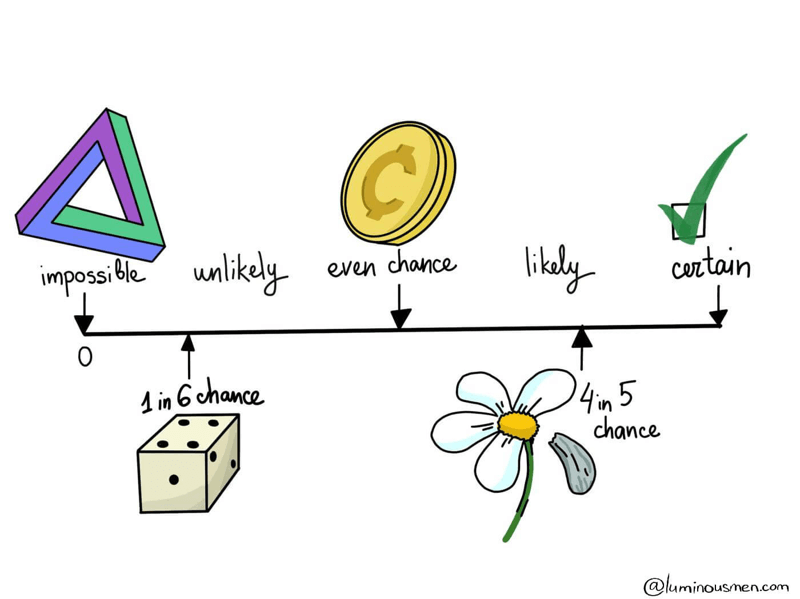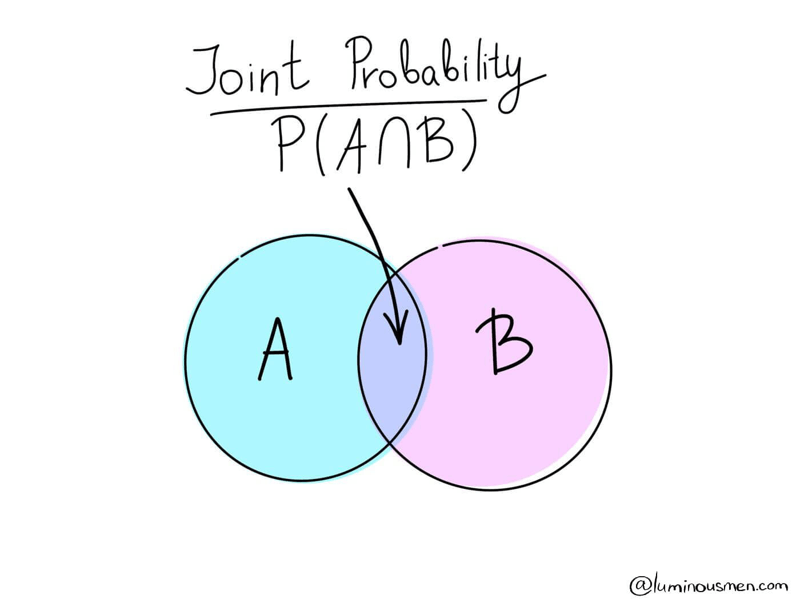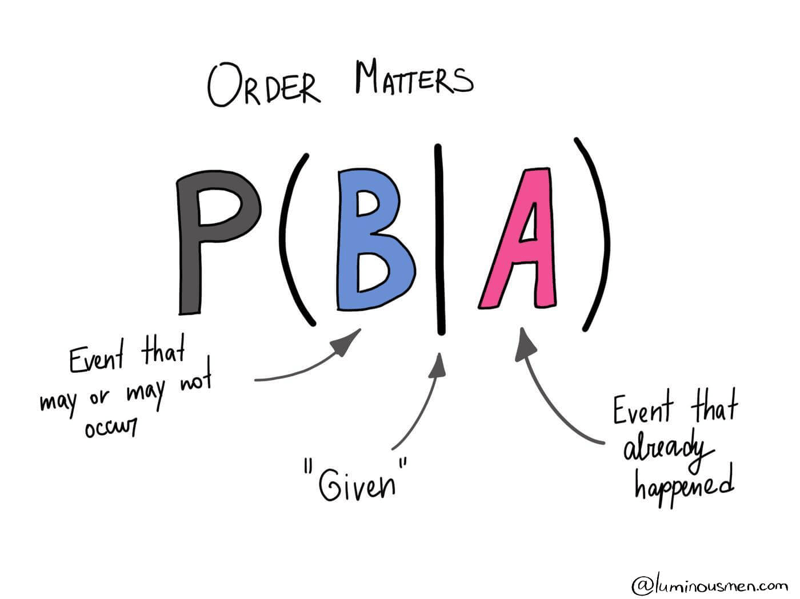There are a lot of engineers who have never been involved in the field of statistics or data science. But in order to build a data science pipelines or rewrite produced code by data scientists to an adequate, easily maintained code many nuances and misunderstandings arise from the engineering side. For those Data/ML engineers and novice data scientists, I make this series of posts. I'll try to explain some basic approaches in plain English and, based on it, explain some of the Data Science basic concepts.

The whole series:

True logic of this world lies in the calculus of probabilities — James Clerk Maxwell

Data science often uses statistical inferences to predict or analyze insights from data, while statistical inferences use probability and its characteristics. So knowing probability and its applications are important to effectively handle data science problems.

## Events. Probabilities of events

One of the basic concepts in statistics is an event. Events are simply results of experiments. Events can be certain, impossible or random.

A certain event is an event that as a result of an experiment (the execution of certain actions with a certain set of conditions) will occur in 100% cases. For example, a tossed coin will certainly fall.

Impossible event — an event, as the name implies, that will not occur as a result of the experiment. For example, a tossed coin will fly in the sky — this is an 'impossible' event.

And finally, an event is called a random event if the event may or may not occur as a result of the experiment. There should be present fundamental criteria of randomness in such an experiment: a random event is a consequence of random factors which influence cannot be predicted or such predictions can be extremely difficult. For example, as a result of a coin toss, tails will fall out. In this case, random factors are the shape and physical characteristics of the coin, the strength/direction of the throw, air resistance, etc.Let us consider in more detail the flip of a coin (meaning a fair coin — a coin in which both results ("heads and tails") are equally likely). There are 2 mutually exclusive outcomes: heads or tails. The outcome of the flip is random since the observer cannot analyze and take into account all the factors that influence the result. What is the probability of heads? Most answer ½, but why?

Let's name A the event that came up as tails. Let the coin be thrown n times. Then the probability of the event A can be defined as:

The probability of an event happening = number of ways it can happen / Total number of outcomes

This is called the frequency of event A in a long series of tests.

Example: there are 4 Kings in a deck of 52 cards. What is the probability of picking a King?

Number of ways it can happen: 4 (there are 4 Kings)

Total number of outcomes: 52 (there are 52 cards in total)

So the probability = 4/52 = 1/13

It turns out that in various test series the corresponding frequency for large n is fluctuating around a constant value P(A). This value is called the probability of event A and is denoted by the letter P — an abbreviation for Probability.

The probability lies in the range [0, 1], where, in general, 0 indicates the impossibility of the event, and 1 indicates certainty. The higher the probability of an event, the greater the likelihood that an event will occur.

Example: What is the probability of drawing a Jack or a Queen from a well-shuffled deck of 52 cards?

If we have 4 Jack and 4 Queen cards, the probability is simply the sum of the individual probabilities.

Number of ways it can happen: 4 (there are 4 Jacks) and 4 (there are 4 Queens) = 8

Total number of outcomes: 52 (there are 52 cards in total)

So the P(Jack or Queen) = 8/52 = 2/13

## Event types

Two random events A and B are called independent if the occurrence of one of them does not change the probability of the occurrence of the other. Otherwise, events A and B are called dependent.

Knowing that the coin landed on head on the first toss, does not provide any useful information for determining what the coin will land on in the second toss. The probability of a head or a tail on the second toss is 1/2, regardless of the outcome of the first toss. Probabilities of independent events should be multiplied to get the total probability of the occurrence of all of them.

Example: What are the chances of getting heads 3 times in a row?

Let's define possible outcomes of 3 coin tosses(H - heads, T - tails):

HHH, HHT, HTH, THH, TTH, THT, HTT, TTT

Number of ways it can happen: 1

Total number of outcomes: 8

So, the answer is 1/8. However, we know that the results of a coin toss are independent and we can multiply them to get the total probability: P(3x heads) = P(heads)P(heads)P(heads) = 1/2 * 1/2 * 1/2 = 1/8

On the other hand, knowing that the first card drawn from a deck is an ace does provide useful information for calculating the probabilities of consecutive draws. So, the probability of drawing yet another ace is going to be 3 over 51, instead of 4 over 52 because we know that we already remove one of the aces from the deck.

Disjoint  events cannot happen at the same time. A synonym for this term is "mutually exclusive".

For example, the outcome of a single coin toss cannot be a head and a tail, it can be either head or tails.

The non-disjoint event can happen at the same time. Therefore they can overlap, and the probability of overlapping should be excluded from total probability to avoid double counting.

Example: What is the probability of drawing a Jack or a Red card from a well-shuffled deck of 52 cards?

Several ways it can happen: 4 (there are 4 Jacks) and 26 (there are 26 Red cards). But there are 2 Red cards overlap between them. Two red jacks that fit both criteria.

Total number of outcomes: 52 (there are 52 cards in total)

So, P(Jack or Red card) = P(Jack) + P(Red card) - P(Jack and Red card) = 4/52 + 26/52 - 2/52 = 7/13

## Types of probabilitiesJoint probability is a type of probability where more than one event occurring simultaneously. The joint probability is the probability that event A will occur at the same time as event B.

For example, from a deck of 52 cards, the total probability of receiving a red card and 6 is P(6 ∩ red) = 2/52 = 1/26, since there are two red sixes in the deck of cards — six hearts and six diamonds. You can also use the formula to calculate the total probability — P(6 ∩ red) = P(6)P(red) = 4/52 * 26/52 = 1/26.

The symbol "∩" in joint probability is an intersection. The probability of occurrence of an event A and an event B is the same as the intersection of A and B sets. Venn Diagram is perhaps the best visual tool to explain.

Marginal probability — a probability of any single event occurring unconditioned on any other events.

Whenever someone asks you whether the weather is going to be rainy or sunny today(without any conditional or prior information), you are computing a marginal probability.Conditional probability — is a probability of an event given that (by assumption, presumption, assertion or evidence) another event has occurred. When I ask you what is the probability that today will be rainy or sunny given that I noticed the temperature is going to be above 80F, you are computing a conditional probability. There is a specific notation for conditional probability shown in the image above.

So, we want to understand the probability of event B given A. To get the probability of event B occurred we should divide all events that lead to event B by all possible events. In this situation, event A has occurred, so the event that leads to event B are A ∩ B and all possible events are B + A ∩ B. Thus,

$$P(B|A) = \frac{P(A ∩ B)}{P(B + A ∩ B)}$$

Example: A math teacher gave her class two tests. 25% of the class passed both tests and 42% of the class passed the first test. What is the probability of those who passed the first test also passed the second test?

P(Second|First) = P(First and Second) / P(First) = 0.25/0.42 = 0.60 = 60%

## Conclusion

In this post, we got acquainted with the basic concepts of probability and probability algebra. In the next post, we will talk about the Bayes theorem and look at the world through the eyes of Bayes.

Next postBuy me a coffee